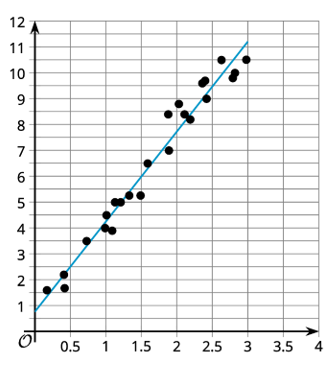# A.3.4.3 Food Markup

The scatter plot shows the sale price of several food items, y, and the cost of the ingredients used to produce those items, x, as well as a line that models the data. The line is also represented by the equation y = 3.48x + 0.67.What is the predicted sale price of an item that has ingredients that cost \$1.50? Explain or show your reasoning.

What is the predicted ingredient cost of an item that has a sale price of \$7? Explain or show your reasoning.

What is the slope of the linear model? What does that mean in this situation?

What is the y-intercept of the linear model? What does this mean in this situation? Does this make sense?

Are the values obtained from looking at the graph close to the values calculated using the equation?